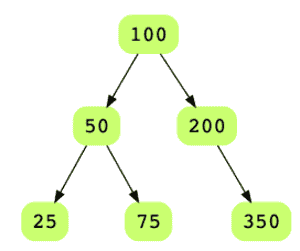# Level Order Traversal of Binary Tree

## Problem Statement

Given the root of a binary tree, display the node values at each level. Node values for all levels should be displayed on separate lines. Let’s take a look at the below binary tree.Level order traversal for this tree should look like:

• 100
• 50, 200
• 25, 75, 350

## Try it yourself

string level_order_traversal(BinaryTreeNode* root) {
string result = "";
//TODO: Write - Your - Code

return result;
}

## Solution

// Using two queues
void level_order_traversal_1(BinaryTreeNode* root) {
if (root == nullptr) {
return;
}

queue<BinaryTreeNode*> queues;

queue<BinaryTreeNode*>* current_queue = &queues;
queue<BinaryTreeNode*>* next_queue = &queues;

current_queue->push(root);
int level_number = 0;

while (!current_queue->empty()) {
BinaryTreeNode* temp = current_queue->front();
current_queue->pop();
cout << temp->data << ",";

if (temp->left != nullptr) {
next_queue->push(temp->left);
}

if (temp->right != nullptr) {
next_queue->push(temp->right);
}

if (current_queue->empty()) {
cout << endl;
++level_number;
current_queue = &queues[level_number % 2];
next_queue = &queues[(level_number + 1) % 2];
}
}
cout << endl;
}

int main(int argc, char* argv[]) {

BinaryTreeNode* root = create_random_BST(15);
level_order_traversal_1(root);
cout << endl;
cout << "Inorder = ";
display_inorder(root);

}

## Solution Explanation

### Runtime Complexity

The runtime complexity of this solution is linear, O(n).

### Memory Complexity

The memory complexity of this solution is linear, O(n).

### Solution Breakdown

Here, you are using two queues: current_queue and next_queue. You push the nodes in both queues alternately based on the current level number.

You’ll dequeue nodes from the current_queue, print the node’s data, and enqueue the node’s children to the next_queue. Once the current_queue becomes empty, you have processed all nodes for the current level_number. To indicate the new level, print a line break (’\n’), swap the two queues, and continue with the above-mentioned logic.

After printing the leaf nodes from the current_queue, swap current_queue and next_queue. Since the current_queue would be empty, you can terminate the loop.

Learn in-demand tech skills in half the time